# Wall Elevations

## Recommended PostsHi, Was wondering if there is a routene available where a 2D room is traced out from a floor plan, and (at another point) we have (in a sequence
) the room floor plan replicated, and the wall elevations and the room reflected ceiling plan placed side by side (once we input the floor height). All in 2D

Could we also point out openings in the original floor plan (like windows and door openings with dimension inputs) which could be cut out in the respective wall elevations drawn ?

This would really facilitate the drawing of elec points / service points at different heights in the room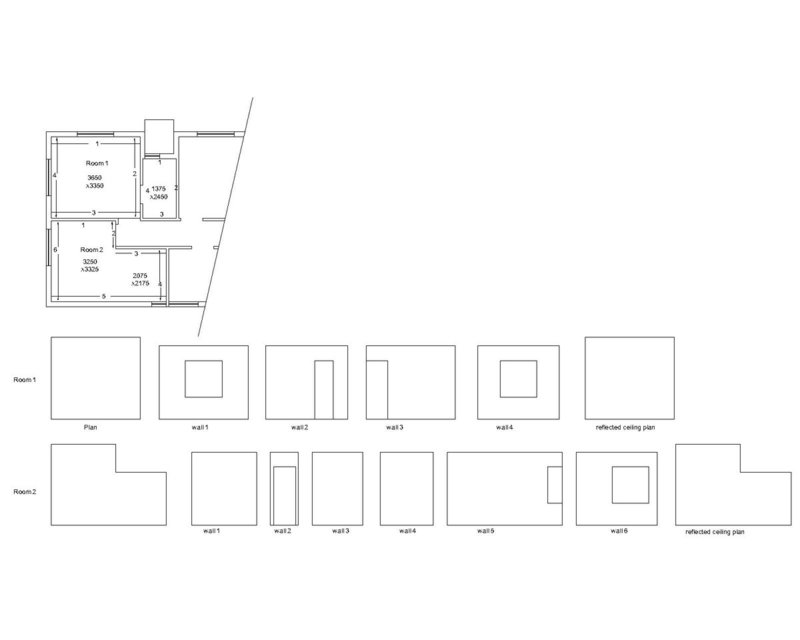##### Share on other sites##### Share on other sitesLike this its 2d. Plain Autocad no solids. Its is an add on which I co authored currently bringing it up to date.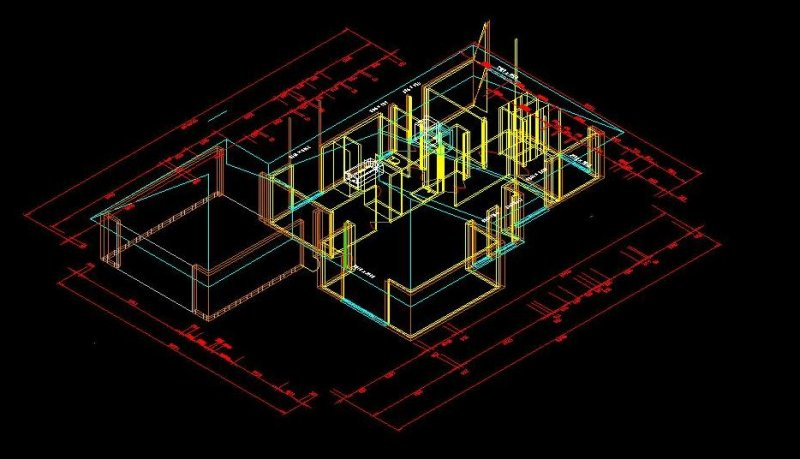##### Share on other sitesI think the OP want

- Run the routine

- The routine asks for floor plan corners

- The routine asks for room height

- The routine draws the plan, reflected plan, each wall in separate.

Is not it?

##### Share on other sitesBased on @asos2000 comment, try this minimally tested :

```(defun c:room-unfold ( / osm p1 p2 wall ans done openings doors windows walls doorh windp windh roomh p room ll ur v dx d )
(setq osm (getvar 'osmode))
(setvar 'osmode 33)
(while (not done)
(setq p1 (getpoint "\nPick or specify first wall corner <ENTER - NO WALLS> : "))
(if (null p1)
(setq done t)
(progn
(initget 1)
(setq p2 (getpoint p1 "\nPick or specify second wall corner : "))
(setq wall (cons (list p1 p2) wall))
(while (not openings)
(initget "Yes No")
(setq ans (getkword "\nWall has openings [Yes/No] <Yes> : "))
(if (null ans)
(setq ans "Yes")
)
(if (= ans "Yes")
(progn
(while (not doors)
(setq p1 (getpoint "\nPick or specify first door anchor point <ENTER - NO DOORS> : "))
(if (null p1)
(setq doors t)
(progn
(initget 1)
(setq p2 (getpoint p1 "\nPick or specify second door anchor point : "))
(setq wall (append wall (list (list "D" p1 p2))))
)
)
)
(while (not windows)
(setq p1 (getpoint "\nPick or specify first window anchor point <ENTER - NO WINDOWS> : "))
(if (null p1)
(setq windows t)
(progn
(initget 1)
(setq p2 (getpoint p1 "\nPick or specify second window anchor point : "))
(setq wall (append wall (list (list "W" p1 p2))))
)
)
)
(setq openings t doors nil windows nil)
)
(setq openings t)
)
)
(setq walls (cons wall walls) openings nil wall nil)
)
)
)
(initget 7)
(setq doorh (getdist "\nPick or specify doors height : "))
(initget 7)
(setq windp (getdist "\nPick or specify windows parapet : "))
(initget 7)
(setq windh (getdist "\nPick or specify windows height : "))
(setq roomh 0.0)
(while (<= roomh (apply 'max (list doorh (+ windp windh))))
(initget 7)
(setq roomh (getdist "\nPick or specify room height : "))
)
(initget 1)
(setq p (getpoint "\nPick or specify insertion point : "))
(setq room (mapcar 'car walls))
(setq ll (list (apply 'min (mapcar 'car (apply 'append room))) (apply 'min (mapcar 'cadr (apply 'append room)))))
(setq ur (list (apply 'max (mapcar 'car (apply 'append room))) (apply 'max (mapcar 'cadr (apply 'append room)))))
(setq v (mapcar '- p ll))
(vl-cmdf "_.PLINE")
(foreach pp (mapcar 'car room)
(vl-cmdf "_non" (mapcar '+ pp v))
)
(vl-cmdf "_C")
(setq dx (/ (- (car ur) (car ll)) 10.0))
(setq p (mapcar '+ v (list (+ (car ur) dx) (cadr ll))))
(foreach wall (reverse walls)
(setq d (apply 'distance (car wall)))
(vl-cmdf "_.RECTANGLE" "_non" p "_non" (mapcar '+ p (list d roomh)))
(foreach opening (cdr wall)
(if (= (car opening) "D")
(vl-cmdf "_.RECTANGLE" "_non" (mapcar '+ p (list (distance (caar wall) (cadr opening)) 0.0)) "_non" (mapcar '+ p (list (distance (caar wall) (caddr opening)) doorh)))
(vl-cmdf "_.RECTANGLE" "_non" (mapcar '+ p (list (distance (caar wall) (cadr opening)) windp)) "_non" (mapcar '+ p (list (distance (caar wall) (caddr opening)) (+ windp windh))))
)
)
(setq p (mapcar '+ p (list (+ d dx) 0.0)))
)
(setq v (mapcar '- p ll))
(vl-cmdf "_.PLINE")
(foreach pp (mapcar 'car room)
(vl-cmdf "_non" (mapcar '+ pp v))
)
(vl-cmdf "_C")
(setvar 'osmode osm)
(princ)
)```

HTH., M.R.

Edited by marko_ribar
•1

##### Share on other sitesIts so easy its called thickness. No code just use property. Can build multi storey with ELEV another hint. But yes the doors, the roofs, the windows, they are added to the walls.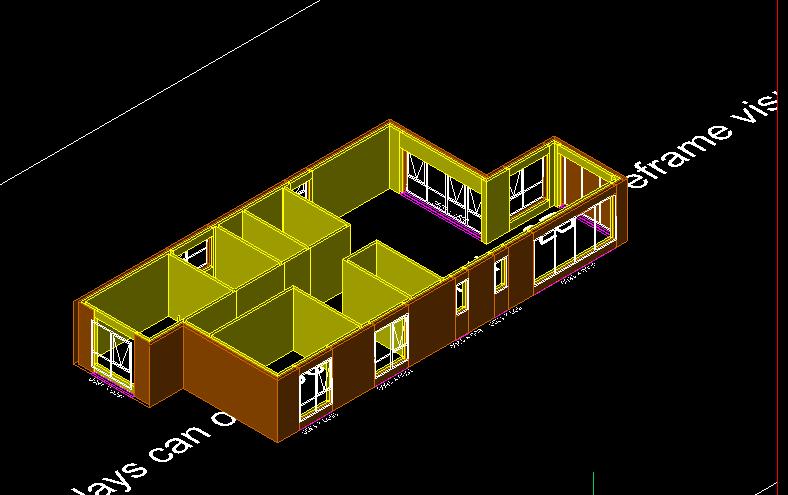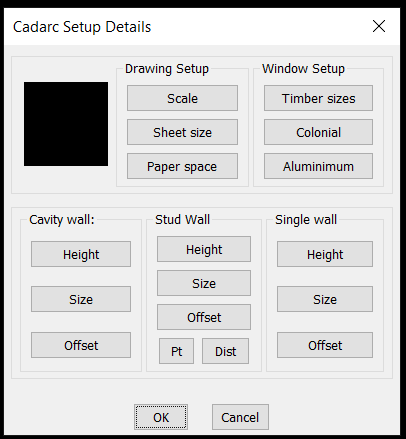##### Share on other sitesApologies for not responding. ..... Thanks for your efforts, I will only be able to try out marko ribar's program tomorrow.

Just a first look at the code, is it possible to draw out the entire room walls in one pline, and then mark out the openings ???? Instead of going wall to wall.... Which would take more time... ??? Or would that be difficult to relate in the code ???

it would just be quicker.....

Bigal, did you mean that no additional software / program would be required for adding thickness to the walls ???? But I feel you would still need to create many sectional views to get the output as is desired (in the sample attached by me)

thanks

##### Share on other sitesI don't know how would you accomplish to mark openings without going wall to wall... Beside that openings may differ with dimensions, so I decided to mod. my code... I think this is more suitable, and yes I waited to see if someone will respond with better approach, but it seems that it would be difficult to do it automatically in few steps without wall to wall... If you find some alternative way to do it, we'll all be grateful to you if you show us how... You could go in 3D as BIGAL showed, but still it is difficult for me to imagine how would you automate it and then again firstly you'd have to model it in 3D... I haven't tried other software, but this is BIM task, don't know maybe BricsCAD or Revit could do it, but I don't know... So long from me, bye...

```(defun c:room-unfold ( / osm pt1 pt2 p1 p2 wall ans done openings ch walls doorh windp windh roomh p room ll ur v dx d ptl k )
(setq osm (getvar 'osmode))
(setvar 'osmode 33)
(while (not done)
(if (null pt1)
(progn
(initget 1)
(setq pt1 (getpoint "\nPick or specify first wall corner : "))
)
)
(initget 1)
(setq pt2 (getpoint pt1 "\nPick or specify second wall corner : "))
(while (equal pt1 pt2 1e-6)
(initget 1)
(setq pt2 (getpoint pt1 "\nPick or specify second wall corner : "))
)
(if (equal pt2 (caar (last walls)) 1e-6)
(setq done t)
)
(setq wall (cons (list pt1 pt2) wall))
(initget "Yes No")
(setq ans (getkword "\nWall has openings [Yes/No] <Yes> : "))
(if (null ans)
(setq ans "Yes")
)
(if (= ans "Yes")
(progn
(setq openings nil)
(while (not openings)
(while (and (setq p1 (getpoint "\nPick or specify first opening anchor point <ENTER - NO MORE OPENINGS> : ")) (not (equal (distance pt1 pt2) (+ (distance pt1 p1) (distance p1 pt2)) 1e-6))))
(if (null p1)
(setq openings t)
(progn
(initget 1)
(setq p2 (getpoint p1 "\nPick or specify second opening anchor point : "))
(while (not (equal (distance pt1 pt2) (+ (distance pt1 p2) (distance p2 pt2)) 1e-6))
(initget 1)
(setq p2 (getpoint p1 "\nPick or specify second opening anchor point : "))
)
(initget "D W")
(setq ch (getkword "\nDoor or Window [D/W] <D> : "))
(if (null ch)
(setq ch "D")
)
(if (= ch "D")
(progn
(if doorh
(progn
(initget 6)
(setq doorh (cond ((getdist (strcat "\nPick or specify door height <" (rtos doorh 2 8) "> : "))) (doorh)))
)
(progn
(initget 7)
(setq doorh (getdist (strcat "\nPick or specify door height : ")))
)
)
(setq wall (append wall (list (list p1 p2 doorh))))
)
(progn
(if windp
(progn
(initget 6)
(setq windp (cond ((getdist (strcat "\nPick or specify window parapet <" (rtos windp 2 8) "> : "))) (windp)))
)
(progn
(initget 7)
(setq windp (getdist (strcat "\nPick or specify window parapet : ")))
)
)
(if windh
(progn
(initget 6)
(setq windh (cond ((getdist (strcat "\nPick or specify window height <" (rtos windh 2 8) "> : "))) (windh)))
)
(progn
(initget 7)
(setq windh (getdist (strcat "\nPick or specify window height : ")))
)
)
(setq wall (append wall (list (list p1 p2 windp windh))))
)
)
)
)
)
)
)
(setq walls (cons wall walls) openings nil wall nil ptl nil pt1 pt2)
(setq ptl (apply 'append (mapcar '(lambda ( x ) (list (car x) (cadr x))) (cdr (car walls)))))
(setq ptl (append (list (caar (car walls))) ptl (list (cadar (car walls)))))
(setq ptl (vl-sort ptl '(lambda ( a b ) (< (distance (car ptl) a) (distance (car ptl) b)))))
(setq ptl (mapcar '(lambda ( a b ) (list a b)) ptl (cdr ptl)))
(setq k 0)
(foreach pair ptl
(if (= (rem (setq k (1+ k)) 2) 1)
(grdraw (car pair) (cadr pair) 1 1)
(progn
(grdraw (car pair) (cadr pair) 3 1)
(setq walls (subst (subst (append (list (car pair) (cadr pair)) (cddr (nth (/ k 2) (car walls)))) (nth (/ k 2) (car walls)) (car walls)) (car walls) walls))
)
)
)
)
(setq roomh 0.0)
(while (<= roomh (apply 'max (mapcar '(lambda ( x ) (if (= (length x) 3) (caddr x) (+ (caddr x) (cadddr x)))) (apply 'append (mapcar 'cdr walls)))))
(initget 7)
(setq roomh (getdist "\nPick or specify room height : "))
)
(initget 1)
(setq p (getpoint "\nPick or specify insertion point : "))
(setq room (mapcar 'car walls))
(setq ll (list (apply 'min (mapcar 'car (apply 'append room))) (apply 'min (mapcar 'cadr (apply 'append room)))))
(setq ur (list (apply 'max (mapcar 'car (apply 'append room))) (apply 'max (mapcar 'cadr (apply 'append room)))))
(setq v (mapcar '- p ll))
(vl-cmdf "_.PLINE")
(foreach pp (mapcar 'car room)
(vl-cmdf "_non" (mapcar '+ pp v))
)
(vl-cmdf "_C")
(setq dx (/ (- (car ur) (car ll)) 10.0))
(setq p (mapcar '+ v (list (+ (car ur) dx) (cadr ll))))
(foreach wall (reverse walls)
(setq d (apply 'distance (car wall)))
(vl-cmdf "_.RECTANGLE" "_non" p "_non" (mapcar '+ p (list d roomh)))
(foreach opening (cdr wall)
(if (= (length opening) 3)
(vl-cmdf "_.RECTANGLE" "_non" (mapcar '+ p (list (distance (caar wall) (car opening)) 0.0)) "_non" (mapcar '+ p (list (distance (caar wall) (cadr opening)) (caddr opening))))
(vl-cmdf "_.RECTANGLE" "_non" (mapcar '+ p (list (distance (caar wall) (car opening)) (caddr opening))) "_non" (mapcar '+ p (list (distance (caar wall) (cadr opening)) (+ (caddr opening) (cadddr opening)))))
)
)
(setq p (mapcar '+ p (list (+ d dx) 0.0)))
)
(setq v (mapcar '- p ll))
(vl-cmdf "_.PLINE")
(foreach pp (mapcar 'car room)
(vl-cmdf "_non" (mapcar '+ pp v))
)
(vl-cmdf "_C")
(setvar 'osmode osm)
(princ)
)```

Edited by marko_ribar

##### Share on other sitesI have Briscad so have been testing Cadarc with it, like Marko_ribar it is a package its just not 1 program. You draw outside walls add internals, then add windows and doors by the time you write some form of data file you would have drawn it and see any errors so could undo. As its 3d your elevations are generated.

##### Share on other sitesI have no knowledge of visual lisp..... and i used to write Autolisp code when i was much much younger....

however, i have tried again.... do suggest if it is ok.

```(defun c:Wallele ()
; get the room corner points
(setvar "cmdecho" 0)

(setq currentlayer (getvar "clayer"))
;(setq slabthk 150) ; later inputs...
(setq wallht 3000)
(while
(setq pt1 (getpoint "\nEnter Wall Start Point or RETURN when done: "))
(setq pt2 (getpoint pt1 "\Enter Wall End point or RETURN when done : "))
(setq stpt (getpoint pt1 "\Enter Pick point to start the WALL elevation : "))
(setq stpt90 (polar stpt (dtr 90) wallht))
(command "pline" stpt  stpt90 (polar stpt90 (dtr 0) (distance pt1 pt2)) (polar stpt (dtr 0) (distance pt1 pt2)) "c" "")
(setq doorwin (strcase (getstring "\nAre there any Door Window Openings in the wall elevation to be marked out ?? [Yes/No] <Y> :")))
(if (or (= doorwin "Y") (= doorwin ""))
(progn
(while
(setq w1 (getpoint "\nPick Opening corner in wall door or window RETURN when done : "))
(setq w2 (getpoint "\nPick other end of Opening  : ")) ; put default value later
(setq w2 (abs (distance w1 w2)))
(setq cillht (getdist "\n Cill height : <1000> "))  ; put default value later
(setq lintelht (getdist "\n Lintel height : <2150>")) ; put default value later
(setq w1x (polar stpt (dtr 0) (distance pt1 w1)))
(setq w2x (polar w1x (dtr 90) cillht ))
(setq w3x (polar w1x (dtr 90) lintelht ))
(setvar "osmode" 0)
(command "pline" w2x w3x (polar w3x (dtr 0) w2) (polar w2x (dtr 0) w2) "c")
(setvar "osmode" 183)

))) ; end while progn if

);end while

) ; end defun```

i just dont know how to put default values in the Cill and Lintel height areas. Furthermore if the points are picked in the wrong order it makes a mess.

I couldnt manage to do the entire room in one go, and as marko-ribar had suggested, i did it one wall at a time... slower, but i didnt have a choice

##### Share on other sitesdo share a link if its available. Thanks

##### Share on other sites##### Share on other sitesFor any one Cadarc was written around 1990+ prior to Autocad Architectural to fill a hole in simple housing & commercial, it was a product by Cad Technology Australia. I have rights to it so Private mail me and I can provide further details, it is not free but is very cheap. Obviously Revit is the way to go now. But at the smaller unique  drafting its a useful tool with to many options to list here.

CADARC 300+ files,  120 lisp files, 117 dwg sample and program blocks, multiple dcl and so on it was not a couple of days works.

For Hanson select corner distance from corner enter window details multi pane etc and it punches hole in wall ie at correct elev in wall, note elev can be multi storey as well.

Edited by BIGAL

##### Share on other sitesdevitg, attaching the sample file

##### Share on other siteshave updated my (lengthy) code....... with some more silly features. still inefficient of course, but that thanks to my lack of prowess at autolisp.

```;************************* function to make unfolded wall elevations ******************
;				Harsh Sanon - 8 Feb 2020
;
;**************************************************************************************

(defun c:Wallele ()
(setvar "cmdecho" 0)

(setq currentlayer (getvar "clayer"))
(setq slabthk (getstring "\n Specify slab thickness : <150> : "))
(if (= slabthk "") (setq slabthk 150)(setq slabthk (atoi slabthk )))
(setq beamdepth (getstring "\n Specify Beam Depth : <600> : "))
(if (= beamdepth "") (setq beamdepth 600)(setq beamdepth (atoi beamdepth )))
(setq beamdepth  (- beamdepth slabthk))
(setq wallht (getstring "\n Specify floor to floor height : <3050> : "))
(if (= wallht "") (setq wallht 3050)(setq wallht (atoi wallht)))
(setq wallht (- wallht slabthk))

(while
(setq pt1 (getpoint "\nEnter Wall Start Point or RETURN when done: "))
(setq pt2 (getpoint pt1 "\Enter Wall End point or RETURN when done : "))
(setq stpt (getpoint pt1 "\Enter Pick point to start the WALL elevation : "))
(setq stpt90 (polar stpt (dtr 90) wallht))
(setq stpt900 (polar stpt90 (dtr 0)  (distance pt1 pt2)))
(setvar "osmode" 0)
(command "pline" stpt  stpt90 (polar stpt90 (dtr 0) (distance pt1 pt2)) (polar stpt (dtr 0) (distance pt1 pt2)) "c" )
(command "pline" stpt90  (polar stpt90 (dtr 90) slabthk)   (polar stpt900 (dtr 90) slabthk) stpt900 "c")
(command "chprop" "l" "" "LT" "DASHED" "")
(setq bmpt (polar stpt90 (dtr 270) beamdepth ))
(command "pline" bmpt (polar bmpt (dtr 0) (distance pt1 pt2)) "")
(command "chprop" "l" "" "LT" "DASHED" "")
(setvar "osmode" 183)
;****
(setq doorwin (strcase (getstring "\nAre there any Door Window Openings in the wall elevation to be marked out ?? [Yes/No] <Y> :")))
(if (or (= doorwin "Y") (= doorwin ""))
(progn
(while
(setq w1 (getpoint "\nPick Opening corner in wall door or window RETURN when done : "))
(setq w2 (getpoint "\nPick other end of Opening  : ")) ; put default value later
(setq w2 (abs (distance w1 w2)))
(setq cillht (getstring "\n Specify Cill Height (0 for Door openings) : <1000> : "))
(if (= cillht "") (setq cillht 1000)(setq cillht (atoi cillht )))
(setq lintelht (getstring "\n Specify LinteL Height : <upto beam> : "))
(if (= lintelht "") (setq lintelht (- wallht beamdepth))  (setq lintelht (atoi lintelht )))
(setq w1x (polar stpt (dtr 0) (distance pt1 w1)))
(setq w2x (polar w1x (dtr 90) cillht ))
(setq w3x (polar w1x (dtr 90) lintelht ))
(setvar "osmode" 0)
(command "pline" w2x w3x (polar w3x (dtr 0) w2) (polar w2x (dtr 0) w2) "c")
(command "line" w2x (polar w3x (dtr 0) w2) "")   (command "mirror" "l" "" (polar w2x (dtr 0) (/ w2 2)) (polar w3x (dtr 0) (/ w2 2)) "" "")
(setvar "osmode" 183)
)  )  ) ; end while progn if

(setq txyn (strcase (getstring "\nWould you like to Label the Completed Wall ?? [Yes/No] <Y> :")))
(if (or (= txyn "Y") (= txyn ""))
(progn
(setq wallname (getstring "\n Wall name to be Labelled : "))
(command "text" "j" "C" (polar pt1 (angle pt1 pt2) (/ (distance pt1 pt2) 2)) (angle pt1 pt2) wallname )
(command "text" "j" "C" (polar stpt (dtr 0) (/ (distance pt1 pt2) 2)) (dtr 0) wallname )
(command "move" "l" "" "@" "@300<270")
)) ; end progn if
);end while

) ; end defun```

##### Share on other siteshanson save you hours of work some hints use Mline with thickness, use copy then rotate3d, this will stand up your walls and you maintain your original 3d structure. Cadarc just uses the fact that as its 3d just change view direction so elevations are auto made at scale in a layout. Roofs are 3d widows are transparent so can see inside if wanted.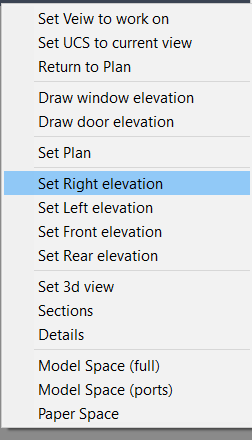##### Share on other sitesBIGAL,

Just a remark for your attached pic., There are cases when there are more than 4 walls : walls could spread at any angle until they form closed room area. The fact is that openings in them are aligned with them and therefore elevations are perpendicular views of them all... So your example is just simple ordinary case of rectangular room, and in reality this is not always true, even OP's example shows that although all walls orthogonal that there can be more than 4 walls - look at lower room (it's kind of like "L" shaped)... I don't know if soft. you are presenting can achieve this sort of presentation, but like I said there can be more than 4 elevations and with walls spreading at any angle (you have to Set angled elevation instead of shown Right/Left/Front/Rear)...

##### Share on other sitesMarko the walls can be any angle and you could draw a pentagon if you want, the default elevations you are correct are the 4 views for simplicity. But as its 2d+ so view from any angle. Its an option. Image very simple example. What about a curved wall ? Before you ask windows would have to be done as facets on the curve. You can punch the window through the wall or not depends on the situation required if no 3d renders required etc

The user manual is 84 pages and yes I have added more options so needs updating.

Will try to find the 3d animations includes the walk from ground up the 3d spiral staircase to 1st floor.

Edited by BIGAL

##### Share on other sitesOn 2/10/2020 at 10:04 PM, marko_ribar said:

I don't know how would you accomplish to mark openings without going wall to wall... Beside that openings may differ with dimensions, so I decided to mod. my code... I think this is more suitable, and yes I waited to see if someone will respond with better approach, but it seems that it would be difficult to do it automatically in few steps without wall to wall... If you find some alternative way to do it, we'll all be grateful to you if you show us how... You could go in 3D as BIGAL showed, but still it is difficult for me to imagine how would you automate it and then again firstly you'd have to model it in 3D... I haven't tried other software, but this is BIM task, don't know maybe BricsCAD or Revit could do it, but I don't know... So long from me, bye...

```
(defun c:room-unfold ( / osm pt1 pt2 p1 p2 wall ans done openings ch walls doorh windp windh roomh p room ll ur v dx d ptl k )
(setq osm (getvar 'osmode))
(setvar 'osmode 33)
(while (not done)
(if (null pt1)
(progn
(initget 1)
(setq pt1 (getpoint "\nPick or specify first wall corner : "))
)
)
(initget 1)
(setq pt2 (getpoint pt1 "\nPick or specify second wall corner : "))
(while (equal pt1 pt2 1e-6)
(initget 1)
(setq pt2 (getpoint pt1 "\nPick or specify second wall corner : "))
)
(if (equal pt2 (caar (last walls)) 1e-6)
(setq done t)
)
(setq wall (cons (list pt1 pt2) wall))
(initget "Yes No")
(setq ans (getkword "\nWall has openings [Yes/No] <Yes> : "))
(if (null ans)
(setq ans "Yes")
)
(if (= ans "Yes")
(progn
(setq openings nil)
(while (not openings)
(while (and (setq p1 (getpoint "\nPick or specify first opening anchor point <ENTER - NO MORE OPENINGS> : ")) (not (equal (distance pt1 pt2) (+ (distance pt1 p1) (distance p1 pt2)) 1e-6))))
(if (null p1)
(setq openings t)
(progn
(initget 1)
(setq p2 (getpoint p1 "\nPick or specify second opening anchor point : "))
(while (not (equal (distance pt1 pt2) (+ (distance pt1 p2) (distance p2 pt2)) 1e-6))
(initget 1)
(setq p2 (getpoint p1 "\nPick or specify second opening anchor point : "))
)
(initget "D W")
(setq ch (getkword "\nDoor or Window [D/W] <D> : "))
(if (null ch)
(setq ch "D")
)
(if (= ch "D")
(progn
(if doorh
(progn
(initget 6)
(setq doorh (cond ((getdist (strcat "\nPick or specify door height <" (rtos doorh 2 8) "> : "))) (doorh)))
)
(progn
(initget 7)
(setq doorh (getdist (strcat "\nPick or specify door height : ")))
)
)
(setq wall (append wall (list (list p1 p2 doorh))))
)
(progn
(if windp
(progn
(initget 6)
(setq windp (cond ((getdist (strcat "\nPick or specify window parapet <" (rtos windp 2 8) "> : "))) (windp)))
)
(progn
(initget 7)
(setq windp (getdist (strcat "\nPick or specify window parapet : ")))
)
)
(if windh
(progn
(initget 6)
(setq windh (cond ((getdist (strcat "\nPick or specify window height <" (rtos windh 2 8) "> : "))) (windh)))
)
(progn
(initget 7)
(setq windh (getdist (strcat "\nPick or specify window height : ")))
)
)
(setq wall (append wall (list (list p1 p2 windp windh))))
)
)
)
)
)
)
)
(setq walls (cons wall walls) openings nil wall nil ptl nil pt1 pt2)
(setq ptl (apply 'append (mapcar '(lambda ( x ) (list (car x) (cadr x))) (cdr (car walls)))))
(setq ptl (append (list (caar (car walls))) ptl (list (cadar (car walls)))))
(setq ptl (vl-sort ptl '(lambda ( a b ) (< (distance (car ptl) a) (distance (car ptl) b)))))
(setq ptl (mapcar '(lambda ( a b ) (list a b)) ptl (cdr ptl)))
(setq k 0)
(foreach pair ptl
(if (= (rem (setq k (1+ k)) 2) 1)
(grdraw (car pair) (cadr pair) 1 1)
(progn
(grdraw (car pair) (cadr pair) 3 1)
(setq walls (subst (subst (append (list (car pair) (cadr pair)) (cddr (nth (/ k 2) (car walls)))) (nth (/ k 2) (car walls)) (car walls)) (car walls) walls))
)
)
)
)
(setq roomh 0.0)
(while (<= roomh (apply 'max (mapcar '(lambda ( x ) (if (= (length x) 3) (caddr x) (+ (caddr x) (cadddr x)))) (apply 'append (mapcar 'cdr walls)))))
(initget 7)
(setq roomh (getdist "\nPick or specify room height : "))
)
(initget 1)
(setq p (getpoint "\nPick or specify insertion point : "))
(setq room (mapcar 'car walls))
(setq ll (list (apply 'min (mapcar 'car (apply 'append room))) (apply 'min (mapcar 'cadr (apply 'append room)))))
(setq ur (list (apply 'max (mapcar 'car (apply 'append room))) (apply 'max (mapcar 'cadr (apply 'append room)))))
(setq v (mapcar '- p ll))
(vl-cmdf "_.PLINE")
(foreach pp (mapcar 'car room)
(vl-cmdf "_non" (mapcar '+ pp v))
)
(vl-cmdf "_C")
(setq dx (/ (- (car ur) (car ll)) 10.0))
(setq p (mapcar '+ v (list (+ (car ur) dx) (cadr ll))))
(foreach wall (reverse walls)
(setq d (apply 'distance (car wall)))
(vl-cmdf "_.RECTANGLE" "_non" p "_non" (mapcar '+ p (list d roomh)))
(foreach opening (cdr wall)
(if (= (length opening) 3)
(vl-cmdf "_.RECTANGLE" "_non" (mapcar '+ p (list (distance (caar wall) (car opening)) 0.0)) "_non" (mapcar '+ p (list (distance (caar wall) (cadr opening)) (caddr opening))))
(vl-cmdf "_.RECTANGLE" "_non" (mapcar '+ p (list (distance (caar wall) (car opening)) (caddr opening))) "_non" (mapcar '+ p (list (distance (caar wall) (cadr opening)) (+ (caddr opening) (cadddr opening)))))
)
)
(setq p (mapcar '+ p (list (+ d dx) 0.0)))
)
(setq v (mapcar '- p ll))
(vl-cmdf "_.PLINE")
(foreach pp (mapcar 'car room)
(vl-cmdf "_non" (mapcar '+ pp v))
)
(vl-cmdf "_C")
(setvar 'osmode osm)
(princ)
)```

power full lisp , but , you must improve it to add option to draw arched window and door .......Greetings and peace

##### Share on other sites"You must improve it to add option"

You must remember that code provided is for free so if you want improvements you either wait or pay to have something done quickly, in saying quickly Cad-arc was written over a period of 2 years.

## Join the conversation

You can post now and register later. If you have an account, sign in now to post with your account.
Note: Your post will require moderator approval before it will be visible.×   Pasted as rich text.   Paste as plain text instead

Only 75 emoji are allowed.